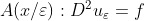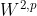## Multiscale & Multilevel Methods

### Registration and Attendance:

The meeting will take place via Zoom, hosted by ICMS .

### Programme:

#### First Session: 12th June, 10:00 - 11:30

9:45-10:00 Meeting opens and opening remarks

10:00-10:45 Daniel Peterseim (Augsburg)
Sparse Compression of Expected Solution Operators [abstract]

10:45-11:30 Jennifer Pestana (Strathclyde)
Preconditioned iterative methods for multilevel Toeplitz matrices [abstract]

11:30-13:30 Break

#### Second Session: 12th June, 13:30 - 15:15

13:30-14:15 Lubomir Banas (Bielefeld)
Finite element approximation of the stochastic total variation flow. [abstract]

14:15-14:45 Timo Sprekeler (Oxford)
Numerical Homogenization of Nondivergence-Form Equations [abstract]

14:45-15:15 Jonna Roden (Edinburgh)
PDE-Constrained Optimization for Multiscale Particle Dynamics [abstract]

Discussion

Abstracts:

Daniel Peterseim, Sparse Compression of Expected Solution Operators
We show that the expected solution operator of prototypical linear elliptic partial differential equations with random coefficients is well approximated by a computable sparse matrix. This result is based on a random localized orthogonal multiresolution decomposition of the solution space that allows both the sparse approximate inversion of the random operator represented in this basis as well as its stochastic averaging. The approximate expected solution operator can be interpreted in terms of classical Haar wavelets. When combined with a suitable sampling approach for the expectation, this construction leads to an efficient method for computing a sparse representation of the expected solution operator which is relevant for stochastic homogenization and uncertainty quantification.

Jennifer Pestana, Preconditioned iterative methods for multilevel Toeplitz matrices
Linear systems involving multilevel Toeplitz matrices arise in a number of applications, e.g., the discretization of certain 2D and 3D partial differential or fractional differential equations, and image debarring problems. Krylov subspace methods are effective solvers for such problems, and for symmetric multilevel Toeplitz matrices theoretical results and efficient methods enable fast solvers with guaranteed convergence rates. Here we describe how to obtain similar results for nonsymmetric multilevel Toeplitz problems by employing a simple symmetrization. We discuss theoretical properties of these symmetrized matrices and propose effective preconditioners.

Lubomir Banas, Finite element approximation of the stochastic total variation flow.
We propose an energy preserving fully discrete finite element approximation of the regularized stochastic total variation flow (STVF) equation. The problem can be interpreted a (regularized) stochastically perturbed gradient flow of the total variation energy functional, which is used, e.g., for image denoising and the modelling of damage evolution. Due to its singular character the solution of the stochastic total variation flow has to be formulated as a stochastic variational inequality (SVI). We show that the numerical solution converges to the SVI solution of the STVF equation for vanishing discretization and regularization parameters. We also present numerical experiments to demonstrate the practicability of the proposed numerical scheme.

Timo Sprekeler, Numerical Homogenization of Nondivergence-Form Equations
In this talk, we will discuss periodic homogenization problems of the formsubject to a homogeneous Dirichlet boundary condition. We derivecorrector estimates and propose, and rigorously analyze, a numerical scheme based on finite element approximations for such problems. If time allows, we extend the results to the case of nonuniformly oscillating coefficients. This is joint work with Yves Capdeboscq (Université de Paris, CNRS, Sorbonne Université, LJLL) and Endre Süli (University of Oxford).

Jonna Roden, PDE-Constrained Optimization for Multiscale Particle Dynamics
There are many industrial and biological processes, such as beer brewing, nano-separation and bird flocking, which can be described by integro-PDEs. These PDEs define the dynamics of a particle density within a fluid bath, under the influence of diffusion, external forces and particle interactions, and often include complex, nonlocal boundary conditions. A key challenge is to optimize these types of processes.  For example, in nano-separation, it is of interest to determine the optimal inflow rate of particles (the control), which leads to high separation of the particles (the target), at a minimal financial cost.  Mathematically, this requires tools from PDE-constrained optimization. A standard technique is to derive a system of optimality conditions and solve it numerically. Due to the nonlinear, nonlocal nature of the governing PDE and boundary conditions, the optimization of multiscale particle dynamics problems requires the development of new theoretical and numerical methods. I will present the system of nonlinear, nonlocal integro-PDEs that describe the optimality conditions for such an optimization problem. Furthermore, I will introduce a numerical method, which combines pseudospectral methods with an optimization algorithm. This provides a tool for the fast and accurate solution of these optimality systems. Finally, some examples of future industrial applications will be given. This is joint work with Ben Goddard and John Pearson.

Local organisers:
Lehel Banjai
Lyonell Boulton
Mariya Ptashnyk

Network organisers:
Agissilaos Athanassoulis
Gabriel Barrenechea
Ben Goddard
Mariya Ptashnyk